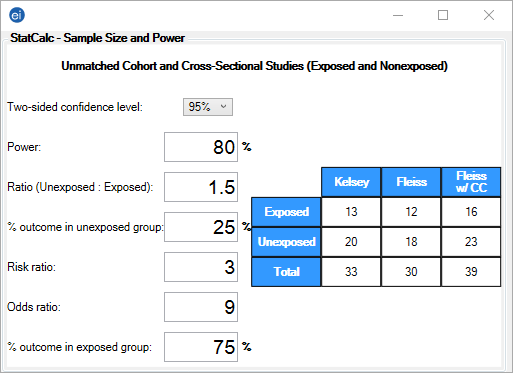# StatCalc: Statistical Calculators

StatCalc

### Cohort and Cross-Sectional

The Cohort and Cross-Sectional study calculates the sample size recommended for a study given a set of parameters and the desired confidence level.

#### Example

The following example demonstrates how to calculate a sample size for a cohort or cross-sectional study. The application will show three different sample size estimates according to three different statistical calculations.

1. From the Epi Info™ main page, select StatCalc.
2. Select Cohort or cross sectional. The Cohort or Cross-Sectional window opens.
3. Select the Two-sided confidence level of 95% from the drop-down list.
4. Enter the desired Power (80%) to detect a group difference at that confidence level.
5. Enter the proportion of unexposed vs. exposed of 1.5. This is a single value and the approximate proportion cannot be entered in the format, # of Unexposed : # of Exposed.
6. Enter the percentage outcome in the unexposed group 25%. This percentage represents the incidence rate of ill patients in the unexposed group
7. Enter the percentage outcome in the exposed group 75%. This percentage represents the incidence rate of ill patients in the exposed group
8. The Risk ratio and Odds ratio fields automatically populate based on the values entered.
9. The output table shows three different estimates of sample size needed.Figure 10.11: Cohort and Cross-Sectional Studies

Page last reviewed: September 16, 2022, 12:00 pm